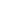Flat 50% off

Ends in

• NEET
•• 11th
•• Physics
• Back to Doubt Clearing

## Please answer the below questionAssertion : An observer is moving due east and wind appears him to blow from north. Then the actual direction of air blow is towards south-east.Reason : Because $\vec V_R = \vec V_A - \vec V_M$ where $\vec V_R$ is the relative velocity of wind with respect to man, $\vec V_A =$ actual velocity of wind (w.r.t. ground) and $\vec V_M =$ velocity of man w.r.t. ground.(a) A(b) B(c) C(d) D

### Asked By Samyuktha RP

Updated Wed, 14 Nov 2018 12:52 pm